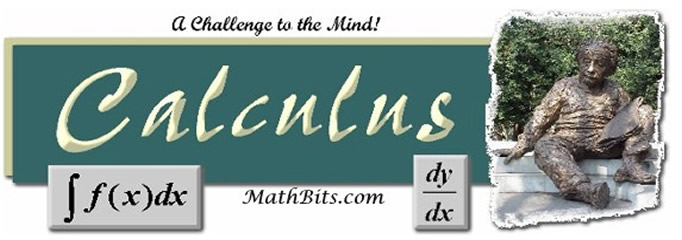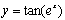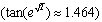Hints for Taking AP Calculus Exam:
 Good Habits for the AP* Exam - From an AP* Exam Grader (excerpts from AP* Calculus reader Doug Shaw - printed with permission) (1.) While units aren't always taken into account, they often are.  Students should get in the habit of always including units when asked, and knowing what the correct units are, even when not asked. (2.)  For some problems, the grader may be instructed NOT to look at any graphs or figures unless the student specifically directs the grader to look at them.  Students should make a point of stating "see my graph below" if they want to illustrate something using a graph, and be aware that a graph sitting by itself does not constitute a mathematical explanation. (3.)  Graphs and figures should always be labeled, or they may not receive full credit.  If a student, for example, draws the graph of g', it should be labeled as g' so it can not be confused with g. (4.)  The students should write their solutions in the appropriate part of their booklets.  If the worst happens, and the student has written the solution in the wrong place, s/he should alert the graders twice.  Once in the place where the solution was written, and once in the place where it should have been written, just to make sure the graders know what happened.  But the safest thing to do is to write the correct solution in the correct place. (5.)  Two words:  RADIAN MODE (6.)  In a multipart problem, where the solution to part a is needed to do part b, it may be to the students' advantage to assume an answer to part a, and use the answer to demonstrate a knowledge of how to do part b.  Perhaps stating it explicitly, "I can't do part a so I am assuming the answer is 0.5 for the purposes of part b.:  This isn't guaranteed to receive credit, but can be a good thing to do if there is time. (7.)  In problems where a descriptive answer is required, the student should make sure to answer the question asked.  The graders are all very experienced teachers, and will not be fooled by a lot of words thrown on the paper in an attempt to obfuscate.  Students' explanations, like their mathematics, should be clear and correct.  For example, if asked to describe what is happening to the temperature over the course of a day, it is better to say "decreasing" than "changing" and it is better to say "decreasing at a constant rate" than "decreasing." (8.)  Students should give numerical approximations three decimal place accuracy.  When plugging numbers into a function, such as pluggingintoit is best to do the rounding at the endthen rounding all alongwhich is not accurate to three decimal places). (9.)  Students should be weaned from over-using the word "it".  Call functions by their names (f(x), g(x), velocity, dy/dx, etc.) (10.) Students do not have to convert temperature given in degrees into radians. (11.) The College Board frowns on "calculatorese".  Statements such as "I entered NumInt(x^2,x,0,1)" are not mathematical explanations. (12.)  The AP Exam is not graded on neatness, but the graders cannot fairly grade work that they cannot read.  Your teacher may be used to your handwriting, but can a stranger read it?